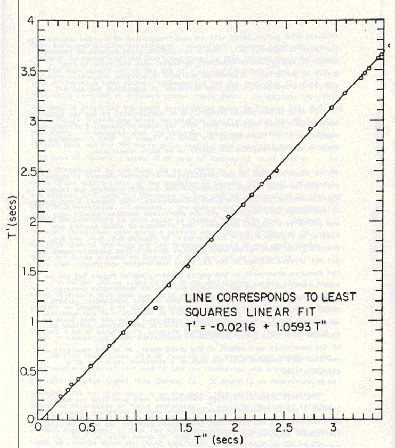Figure 5. Plot of T' versus T" for corresponding characteristics with the values of Table B-1. The marked linearity of the curve demonstrates the validity of the identification. The straight line in the figure is a plot of the given equation, which in turn is a robust linear regression fit to the plotted points. The analysis leading to this figure is given in Appendix B and Table B-1. The point furthest off the line is at T" = 1.195s and is for the incorrectly identified characteristic I, as discussed in Appendix B.

Minimize this window to return.